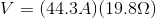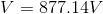# High School Physics : Understanding Voltage in a Circuit

## Example Questions

### Example Question #30 : Electric Circuits

A closed electric circuit is set up so that there is a current of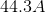and a resistance of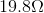. What is the voltage in the circuit?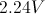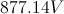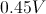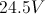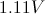Explanation:

For this problem, use Ohm's law: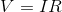.

We are given the current and the resistance. Using these terms, we can solve for the voltage.